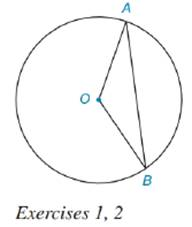Chapter 8.5, Problem 2EElementary Geometry For College St...

7th Edition
Alexander + 2 others
ISBN: 9781337614085

Solutions

Chapter
SectionElementary Geometry For College St...

7th Edition
Alexander + 2 others
ISBN: 9781337614085
Textbook Problem

Given ⊙ O with radii O A - and O B - and chord A B - .a) What type of figure (sector or segment) is bounded by A B - and A B ^ ?b) If A B = 9.7 cm and l A B ^ = 11 . 4 cm, find the perimeter of the figure in (a).To determine

a. To find:

The type of figure bounded by AB- and AB^

Explanation

Segment is the region between chord of a circle and its associated arc.

In the given diagram, AB- is a chord and AB^ is its associated arc. Thus the figure bounded by AB-

To determine

b. To find:

The perimeter of figure in (a).

Still sussing out bartleby?

Check out a sample textbook solution.

See a sample solution

The Solution to Your Study Problems

Bartleby provides explanations to thousands of textbook problems written by our experts, many with advanced degrees!

Get Started

Change 314 to an improper fraction.

Elementary Technical Mathematics

In Exercises 25-30, find the rules for the composite functions f g and g f. 28. f(x)=2x+3;g(x)=x2+1

Applied Calculus for the Managerial, Life, and Social Sciences: A Brief Approach

In Exercises 13 and 14, find the inverse function of f. f(x)=4x+6

Calculus: An Applied Approach (MindTap Course List)